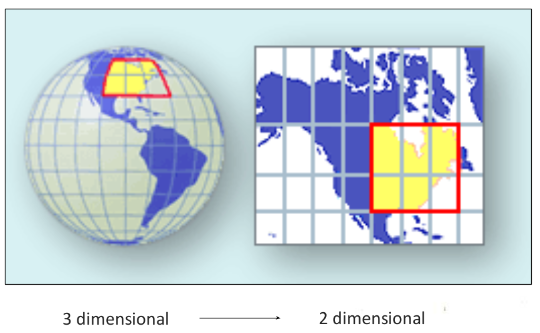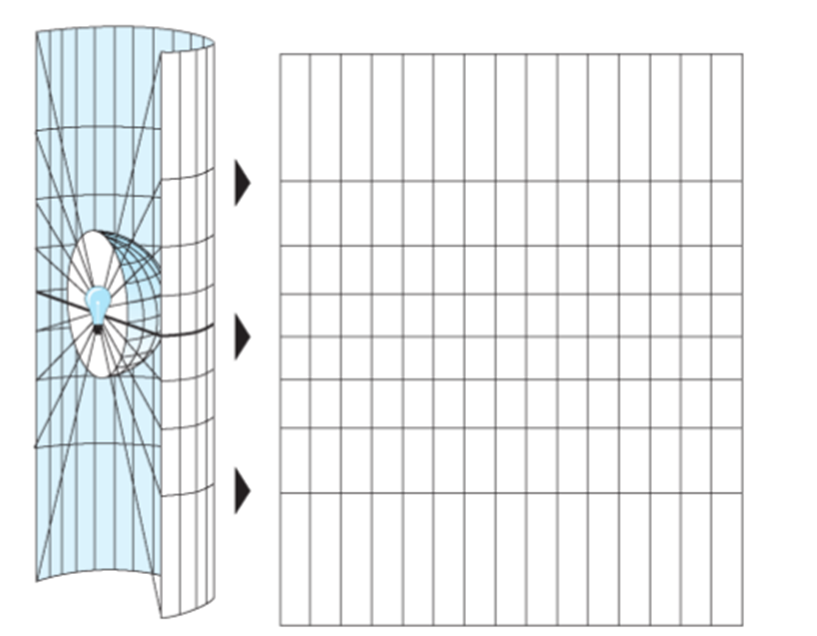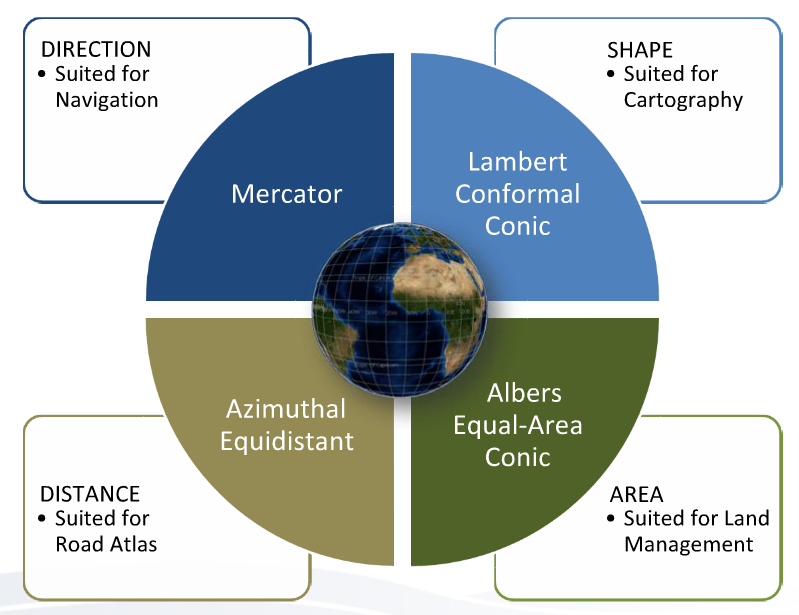﻿ Introduction to SQL and Geospatial Data Processing: Geospatial Queries

# Geospatial Queries

## Overview

Teaching: 15 min
Exercises: 30 min
Questions
• What are the various ways that we can represent geospatial information?

• How does a database store spatial information?

Objectives
• Learn the difference between geographic and projected coordinate systems

• Explore why understanding the coordinate reference frame matters when carrying out basic geospatial analysis

• Become familiar with some of the geospatial toolkits within PostGIS

## Calculating distances

• our last research question was:

What is the most common crime within 5 km of my house?

• To answer this we’ll need to understand something about mapping, and how databases encode spatial information.
• Note that the database currently has latitude and longitude information, for example:
``````SELECT latitude, longitude
FROM seattlecrimeincidents
LIMIT 5;
``````
Latitude Longitude
47.6158384 -122.3181689
47.60087709 -122.3312162
47.59582098 -122.3175691
47.6140991 -122.3174884
47.63148825 -122.3125079

• latitude and longitude are components of the Geographic reference frame and are the most common ways to encode geospatial information:## DISCUSSION: Calculating distances in geographic coordinates

• is it possible for us to calculate straight line distances directly from latitude and longitude data?
• Why or why not?
• Are distances between lines of latitude always the same? Between lines of longitude?

## Map Projections

• often we need to convert a 3D earth to a 2D plane
• this is necessary for accurate calculation of distances, bearing and area calculations• in a general sense, projection is similar to shining a light through a transparent sphere and tracing the lines of lat/long• there are numerous different map projections, each optimized for different types of applications and calculations## Encoding of geospatial information

How does the database currently encode the latitude and longitude information?

• We can determine this by querying the database for information on the type of column variables:
``````SELECT column_name, data_type
FROM information_schema.columns
WHERE table_name = 'seattlecrimeincidents' AND (column_name = 'Latitude' OR column_name = 'Longitude');
``````
column_name data_type
Longitude double precision
Latitude double precision
• we see from this query that the spatial data format is double precision

## PostGIS: a geospatial database extension

• many databases, have extensions that allow for encoding of geospatial information
• in the case of PostgreSQL this extension is called PostGIS
• our sample database already has the PostGIS extension installed and enabled

## Database Geometries

• encoding of geospatial information occurs within a new geometry column:
``````ALTER TABLE seattlecrimeincidents ADD COLUMN geom geometry(Point, 4326);
``````
• this adds an empty geometry column:
Point Latitude Longitude geom
1 47.6158384 -112.3181689
2 47.60087709 -112.3312162

## Spatial Reference System Identifier (SRID)

• `4326` is the SRID for the geographic (latitude/longitude) coordinate system
• SRIDs are a convenient way to keep track of the hundreds of different reference systems
• another label for these is `EPSG` and you can access the list of codes here
``````UPDATE seattlecrimeincidents SET geom = ST_setSRID(ST_MakePoint(longitude, latitude), 4326);
``````
Point Latitude Longitude geom
2 47.60087709 -112.3312162 0101000020E6100000F2B96EA532955EC09A3B5D8AE9CC4740
• the actual content of the geom column is a binary string read by PostGIS
• Now that the database has geometric encoding, we can use a wide range of geospatial functions

## Geospatial functions

• often we receive geospatial information in various different projections and/or coordinate systems
• PostGIS provides mechanisms for us to transform between different systems
• here we will transform our geometries into a projected coordinate system so that we can calculate distances
1. We add a new column that has a projected geometry
• in this case we will use ‘Universal Transverse Mercator Zone 10 North’, which has a SRID of 3717:
`````` ALTER TABLE seattlecrimeincidents
``````
2. Now we carry out the transformation

`````` UPDATE seattlecrimeincidents
SET geom_utm = ST_Transform(geom,3717);
``````
• note that all PostGIS functions begin with `ST` which means “Spatial Tool”
3. Now we can recalculate our distance using a built-in PostGIS distance function:

`````` SELECT ST_Distance(a.geom_utm,b.geom_utm)
FROM seattlecrimeincidents AS a, seattlecrimeincidents AS b
WHERE a.gid=1 AND b.gid=2;
``````

st_distance
1930.45436426609

## Compare results

How does this result compare to the distance we calculated from the latitude/longitude data alone? What explains the difference?

## What is the most common crime within 1 km of my house?

1. Find the coordinates of your house (or some other feature in the Seattle region). HINT: you can use google maps to find the latitude and longitude of any map location
2. Build the SQL query. You will need to nest 4 different functions to do this:
• `ST_MakePoint`: to make a vector point geometry from your lat/long coordinate pair
• `ST_SetSRID`: to tell the database which SRID your lat/long pair conforms to
• `ST_Transform`: to transform your point geomtery to a projected coordinate system (use UTM Zone 10, ‘3717’)
• `ST_Distance`: to calculate the distance between all the geometries in the database and your single point

## Key Points

• All geospatial analysis requires a knowledge of reference frames and coordinate systems

• Be sure to use the correct coordinate system to match your analysis, otherwise you can get unexpected results

• many databases have extensions that encode geospatial information (e.g. PostGIS)

• PostGIS functions provide a wide range of tools to incorporate spatial analysis into your workflow